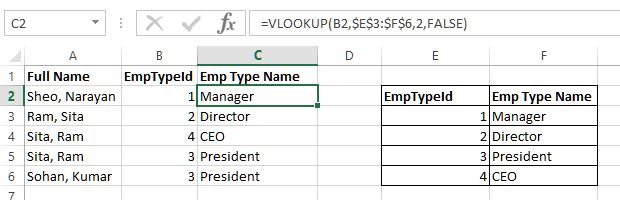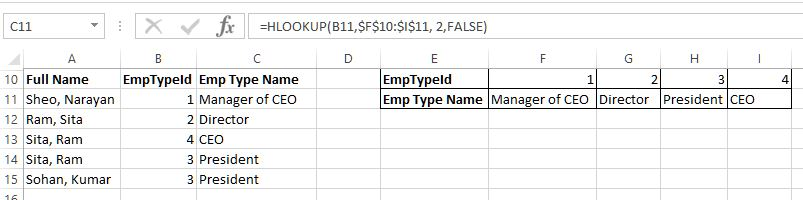# VLookup, HLookup functions in Excel

##### How to work with VLOOKUP & HLOOKUP functions in Excel?

In previous post, we learnt the difference between Find and Search in Excel. In this post, we shall learn about VLOOKUP and HLOOKUP functions in Excel.

### VLOOKUP function

VLOOKUP (Vertical lookup) function is used to look for a value in the main table and return corresponding value from the lookup table.

In this example, we want to write the 'Emp Type Name' in C column based on B column value from the Lookup table from E2 to F6.

The formula in C2 is '=VLOOKUP(B2,\$E\$3:\$F\$6,2,FALSE)' that executes in following way

• 1st parameter - lookup value
• 2nd parameter - lookup table range (notice that the range is in absolute reference)
• 3rd parameter - column index to use to write the value
• 4th parameter - approximate match (TRUE) or exact match (FALSE)
Notice that EmpTypeId = 1 is 'Manager' in the Lookup table so the VLOOKUP function returns 'Manager' in C2 cell.When we copy the same formula to other C cells, we get above result.

### HLOOKUP function

This is almost same as VLOOKUP except the fact that the Lookup table values are horizontally arranged.

In C11 cell, we have '=HLOOKUP(B11,\$F\$10:\$I\$11, 2,FALSE)' formula that does following

• 1st parameter - lookup value
• 2nd parameter - lookup table range (notice that the range is in absolute reference)
• 3rd parameter - row index to use to write the value
• 4th parameter - approximate match (TRUE) or exact match (FALSE)

The EmpTypeId (B11) is 1 so the Lookup table EmpTypeId = 1 is 'Manager of CEO' so we have this in the C11 cell.When we copy the same formula to other C cells, we get above result.

Views: 4834 | Post Order: 30